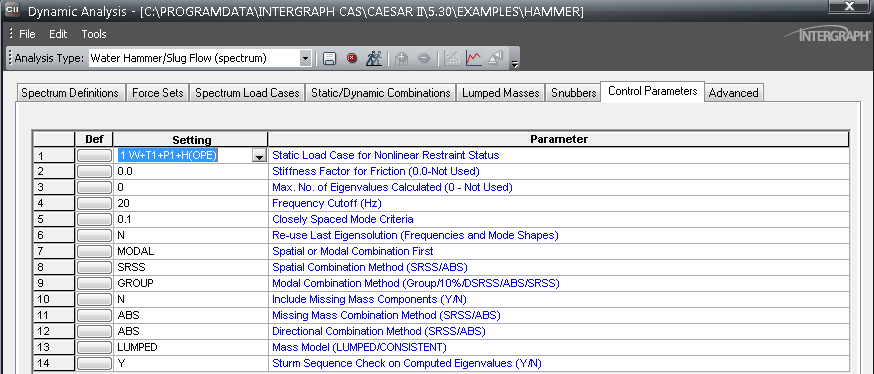# Dynamic Analysis of Water Hammer Loads (HAMMER) - CAESAR II - Reference Data

## CAESAR II Applications Guide

PPMProduct
CAESAR II
PPMCategory_custom
Reference Data
Version_CAESAR
12

In this example, the cooling water supply line shown below suffers a pressure surge when the turbine driven pump drops offline due to a bearing temperature problem. The elbow at node 45 is observed to jump 6 to 8 inches in the X-direction when the turbine trip occurs. To eliminate the large field displacements associated with the turbine trip, an alternative support scheme must be designed.

• Fluid Properties - 250 psi @ 140° F

• Flow Velocity - 6 fps

• Water Bulk Modulus - 313000 psi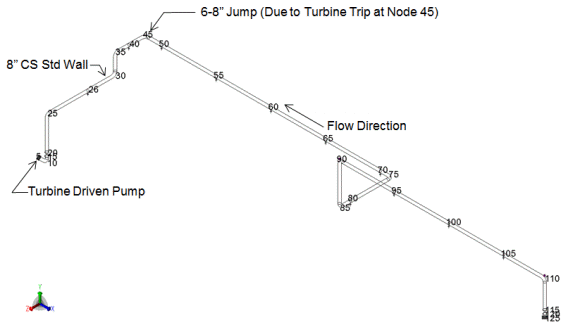SOLUTION

The magnitude of the pump supply side pressure wave, which emanates from the pump discharge at node 5, can be estimated from

dp = r c dv

Where:

dp = the pressure rise due to the pump’s instantaneous stopping

r = the fluid density

c = the speed of sound in the fluid

dv = the change in velocity of the fluid

The speed of sound in the fluid can be estimated from:

c = [Ef/(r+ r(Ef/E)(d/t))]0.5

Where:

Ef = the bulk modulus of the fluid (313000 psi)

E = the modulus of elasticity of the pipe (30E6 psi)

d = the pipe mean diameter

t = the pipe wall thickness

r = the fluid density (62.4 lbm/ft3)

ρ + ρ(Ef/E)(d/t) = 62.4 lbm/ft3

[1 + (313000/30E6)(8.62 -0.322)/0.322] = 79.1875 lbm/ft3

c = (313000 lbf/in2)(ft3/79.1875 lbm)(32.2 lbm ft/lbf sec2)(144in2/ft2)1/2 = 4281 ft/sec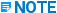For a more detailed discussion and evaluation of the speed of sound, see Piping Handbook, Crocker & King, Fifth Edition, McGraw-Hill pages 3-189 through 3-191

Apply the previously mentioned equation for the magnitude of the water hammer pressure wave.

dp = r c dv = (62.4 lbm/ft3)(4281 ft/sec)(6.0 ft/sec)

= (62.4 lbm/ft3)(4281 ft/sec)(6.0 ft/sec)(lbf sec2/32.2 lbm ft)(ft2/144 in2)

= 345.6 psi

There are two distinct pressure pulses generated when a flowing fluid is brought to a stop. One pulse originates at the supply side of the pump, and the other pulse originates at the discharge side of the pump. This example only deals with the supply side water hammer effect, but the magnitude and impact of the discharge side water hammer load should likewise be investigated when in a design mode.

The time history waveform for both types of water hammer pulses is shown as follows: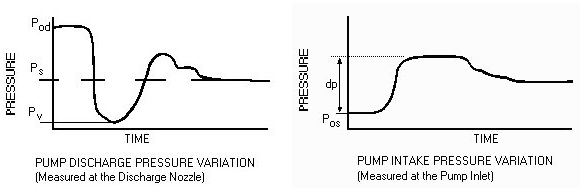Pod - Discharge pressure

Ps - Source (tank or static) pressure

Pos - Suction pressure (while running)

dp - Pressure fluctuation due to the instantaneous stoppage of flow through the pump

Pv - Liquid vapor pressure at flow temperature

There is an unbalanced load on the piping system due to the time it takes the pressure wave to pass successive elbow-elbow pairs. The magnitude of this unbalanced load can be computed from:

F unbalanced = dp x Area

The duration of the load is found from t = L/c, where L is the length of pipe between adjacent elbow-elbow pairs. For this example, the elbow-elbow pairs most likely to cause the large deflections at node 45 are nodes 45-75 and nodes 90-110.

The rise time for the unbalanced dynamic loading should be obtained from the pump manufacturer or from testing, and it can be determined from graphs such as those shown above. For this example, a rise time of 5 milliseconds is assumed.

CALCULATIONS

L 45-75 = 7 + 4(20) + 4 = 90 ft.

L 90-110 = 3(20) + 15 = 75 ft.

Area = P/4di2; di = 8.625-(2)(0.322) = 7.981 in.

Area = P/4(7.981)2 = 50.0 in2

F unbalanced = dp x Area = (345.6) (50.0) = 17289 lbf

t duration = L/c

= (90)/(4281) = 21 milliseconds, on leg from 45 to 75

= (75)/(4281) = 17.5 milliseconds, on leg from 90 to 110

t rise = 5.0 milliseconds

Because the piping in this example is ductile low carbon steel, the major design variable is the large displacement. The problem is assumed to be solved when the restraint system is redesigned to limit the large displacements due to water hammer without causing any subsequent thermal problem due to over-restraint.

1. Generate the DLF spectrum files as shown in the following examples.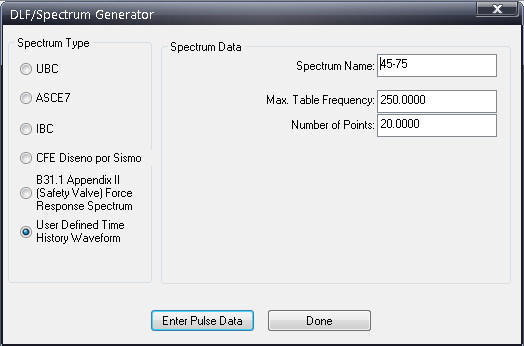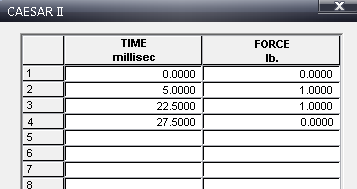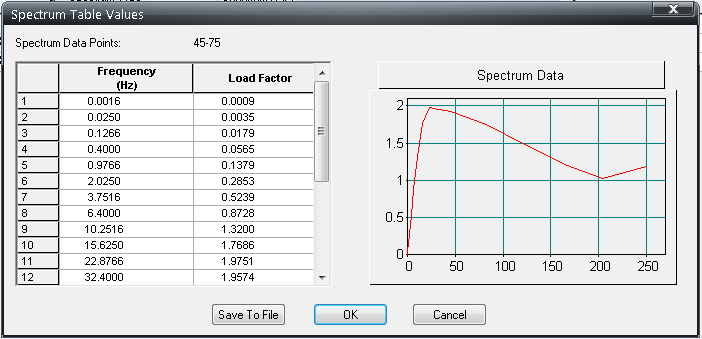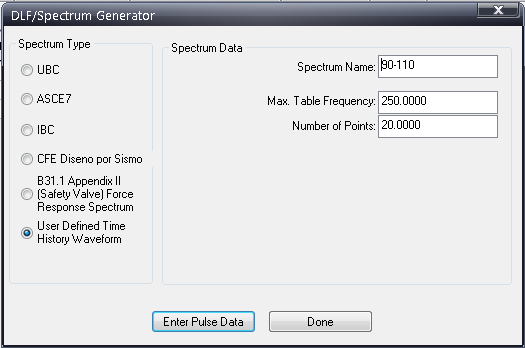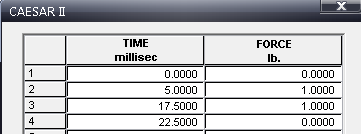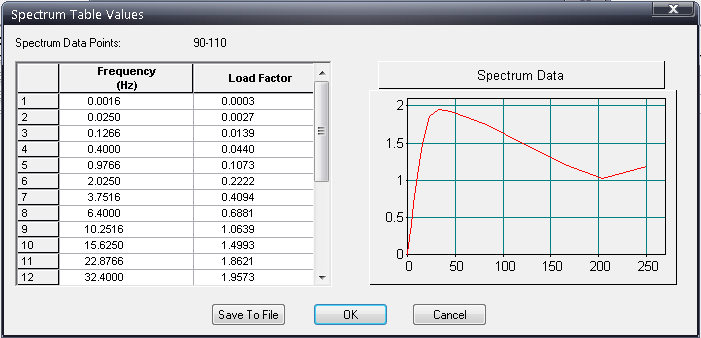2. Define the spectrum on the Spectrum Definitions tab: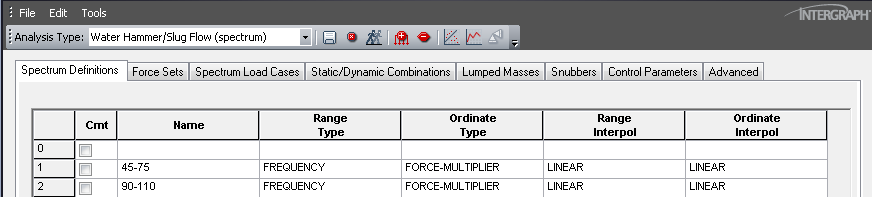3. Define the force sets on the Force Sets tab.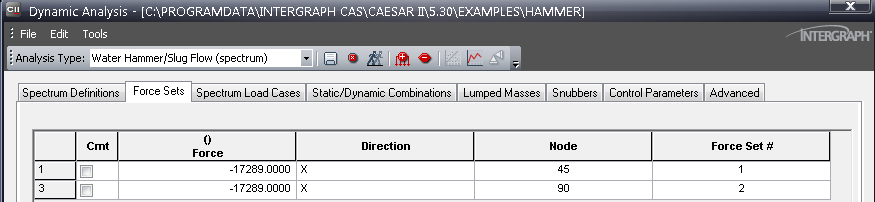Three spectrum load cases are of interest in this example: each spectrum separately and the two of them in combination.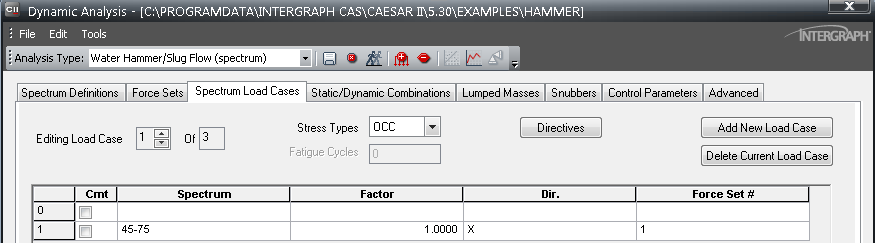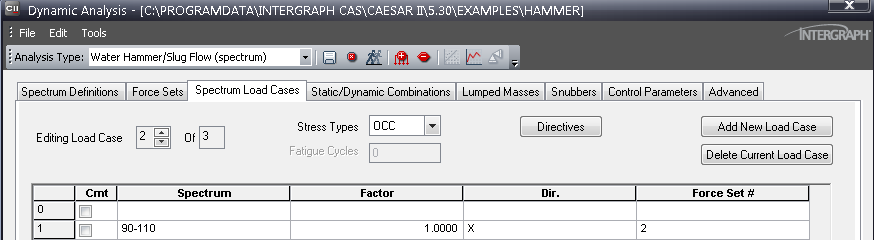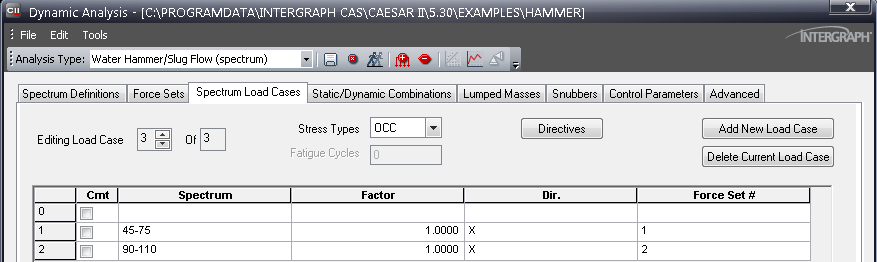The sustained static load case is now combined with each dynamic load case for code stress checks. For operating restraint loads, the static operating case is combined with each dynamic load case, if necessary.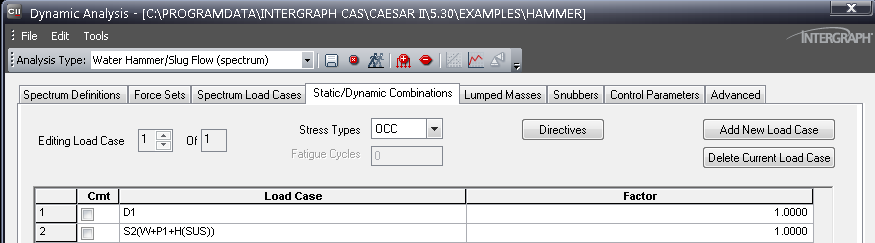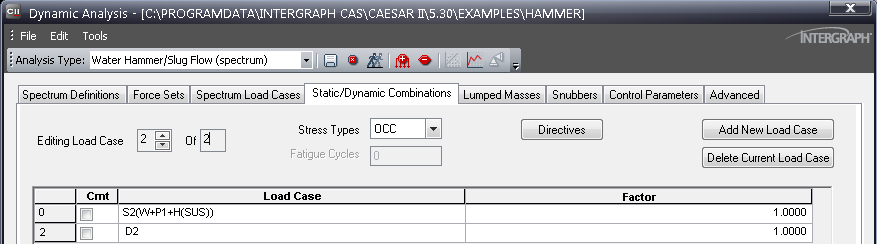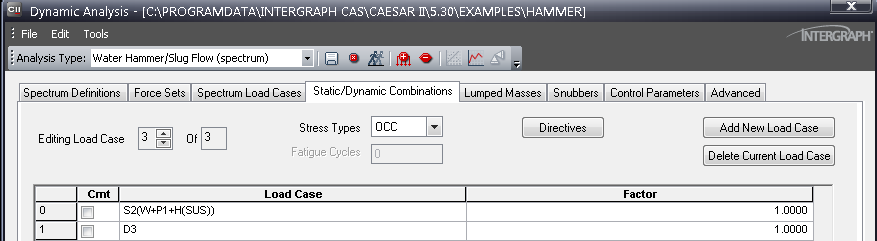4. Set the options on the Control Parameters tab as shown below: